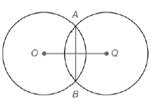Chapter 6.1, Problem 19EElementary Geometry For College St...

7th Edition
Alexander + 2 others
ISBN: 9781337614085

Solutions

Chapter
SectionElementary Geometry For College St...

7th Edition
Alexander + 2 others
ISBN: 9781337614085
Textbook Problem

Circles O and Q have the common chord A B ¯ . If A B = 6 , ⊙ O has a radius of length 4 , and ⊙ Q has a radius of length 6 , how long is O Q ¯ ?Exercises 18, 19

To determine

To find:

The length of OQ¯.

Explanation

Given:

Circles O and Q have the common chord AB¯. The circle with center O has a radius of length 4 and the circle with center Q has a radius of length 6. The length of AB is 6.

Theorem used:

A radius that is perpendicular to the chord bisects the chord.

An angle inscribed in a semicircle is a right angle.

If X is a point on AB¯ and A-X-B, then AX¯+XB¯=AB¯.

Calculation:

In the circle O, OA¯ and OB¯ are radii and in circle Q, QA¯ and QB¯ are radii, where OA¯=4 and QA¯=6. Thus we get a kite with diagonals OQ¯ and AB¯.

We know that the diagonals of a kite are perpendicular to each other.

Hence OQ¯ is perpendicular to AB¯.

By the theorem, “A radius that is perpendicular to the chord bisects the chord”, we get OQ¯ bisects AB¯.

Consider, ΔOAQ.

Since AB¯ is bisected by OQ¯ and we have AB=6.

AE=3.

In right ΔOAE,

Still sussing out bartleby?

Check out a sample textbook solution.

See a sample solution

The Solution to Your Study Problems

Bartleby provides explanations to thousands of textbook problems written by our experts, many with advanced degrees!

Get Started

In Exercises 1124, find the indicated limits, if they exist. 17. limx32x35

Applied Calculus for the Managerial, Life, and Social Sciences: A Brief Approach

In problems 5-8, Use a calculator to evaluate the indicated powers. 8.

Mathematical Applications for the Management, Life, and Social Sciences

Other Equations Find all real solutions of the equation. 108. x3x44=0

Precalculus: Mathematics for Calculus (Standalone Book)

Given P=I2R,I=4, and R=2000, find P.

Elementary Technical Mathematics

True or False: If h(x) = g(x) for all x a and limxah(x)=L, then limxag(x)=L.

Study Guide for Stewart's Single Variable Calculus: Early Transcendentals, 8th